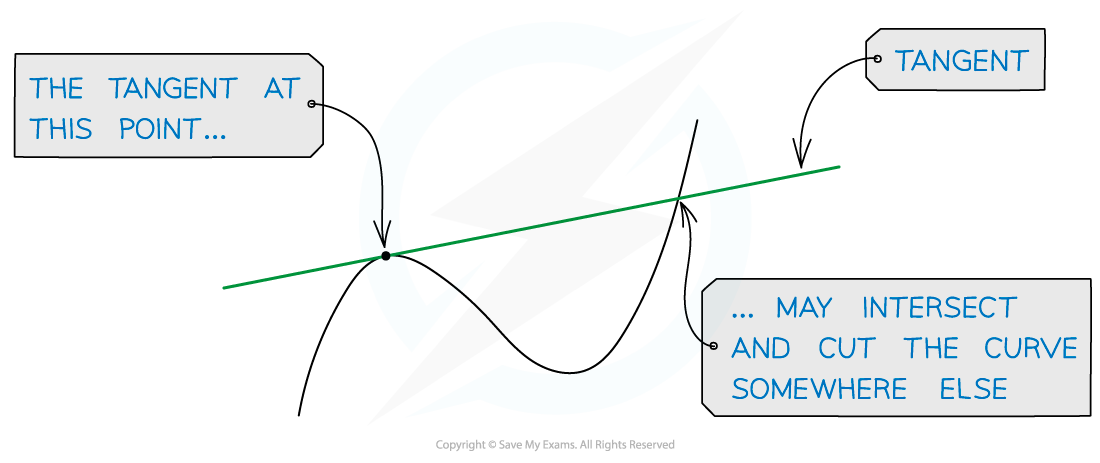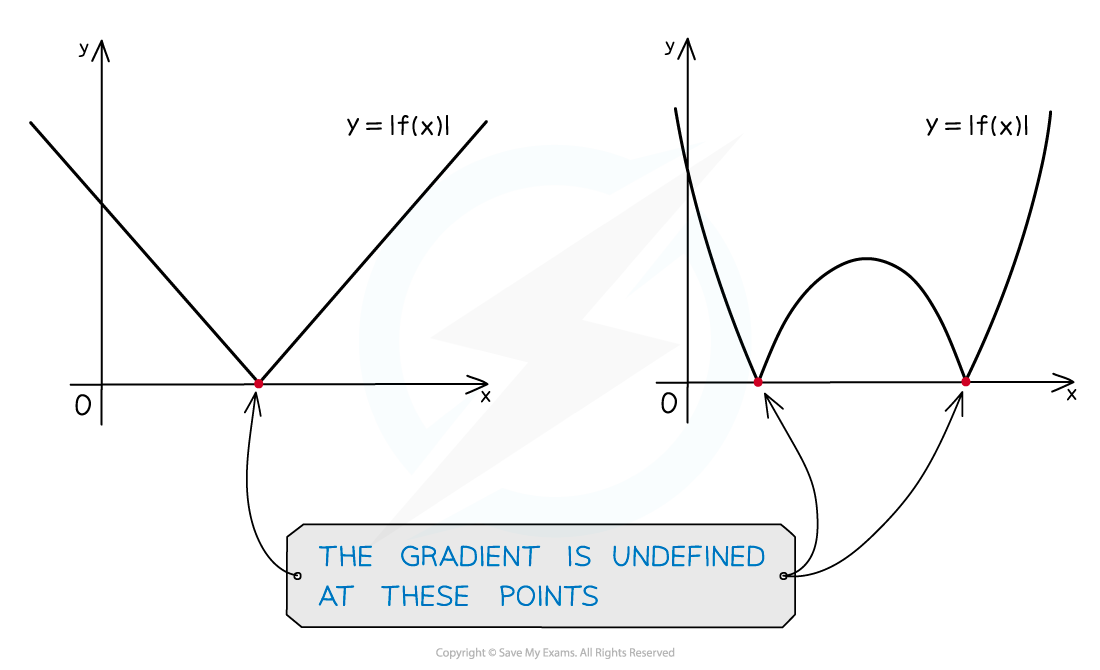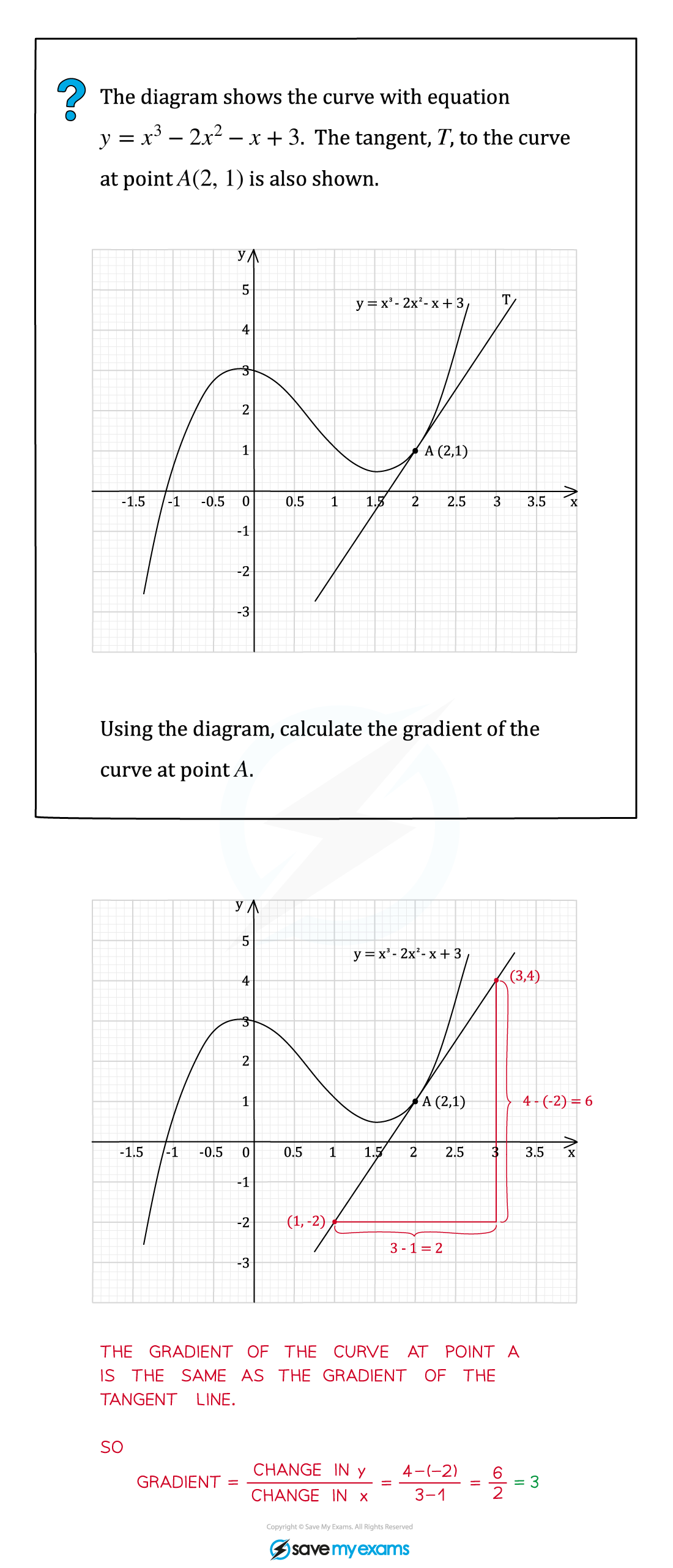# AQA A Level Maths: Pure复习笔记7.1.1 Definition of Gradient

### Definition of Gradient

#### What is the gradient of a curve?

• At a given point the gradient of a curve is defined as the gradient of the tangent to the curve at that point
• A tangent to a curve is a line that just touches the curve at one point but doesn't cut the curve at that point• A tangent may cut the curve somewhere else on the curve• It is only possible to draw one tangent to a curve at any given point
• Note that unlike the gradient of a straight line, the gradient of a curve is constantly changing

#### Exam Tip

• A tangent only exists at points where a curve is smooth.
• For example, there is no tangent (or gradient) at one of the 'corners' in a modulus function.#### Worked Example